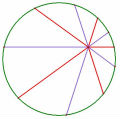極值一題

極值一題

TOP

 老王 老王發短消息 加為好友 當前離線 2# 大 中 小 發表於 2009-9-6 11:15  只看該作者 應該是多項式函數而不是方程式吧 還是用微分 $$f(x)=x^3-3x^2+3kx-3$$ $$f'(x)=3x^2-6x+3k=3(x^2-2x+k)$$ 令 $$f'(x)=0$$的兩根為$$x_1,x_2$$ 由題意 $$\| f(x_1)-f(x_2) \|=32$$ $$x_1^2-2x_1+k=0$$ $$f(x_1)=x_1^3-3x_1^2+3kx_1-3=2kx_1-2x_1+k-3$$ $$f(x_2)=x_2^3-3x_2^2+3kx_2-3=2kx_2-2x_2+k-3$$ 代入得 $$\| 2(k-1)(x_1-x_2) \|=32$$ 又 $$(x_1-x_2)^2=(x_1+x_2)^2-4x_1x_2=4-4k$$ 兩邊平方得 $$4(k-1)^2 \times 4(1-k)=1024$$ $$(1-k)^3=64$$ $$k=-3$$ [ 本帖最後由 老王 於 2009-9-6 11:18 AM 編輯 ] 名豈文章著官應老病休飄飄何所似Essential isolated singularity UID261 帖子308 閱讀權限10 在線時間943 小時 註冊時間2009-5-14 最後登錄2014-3-17  查看詳細資料 TOP
 arend發短消息 加為好友 當前離線 3# 大 中 小 發表於 2009-9-6 15:47  只看該作者 謝謝王老師 在解題過程中,不知要用微分=0的條件 硬作,變的好複雜 謝謝您的指引 arend UID227 帖子375 閱讀權限10 在線時間729 小時 註冊時間2009-2-22 最後登錄2019-6-2  查看詳細資料 TOP
﻿